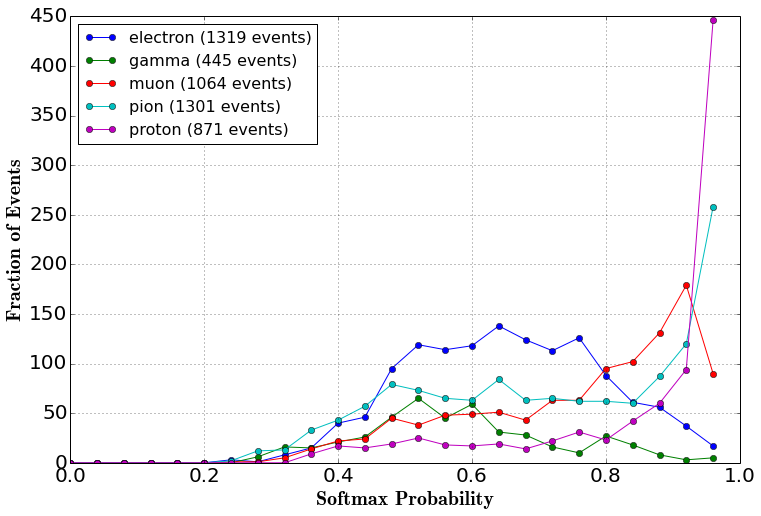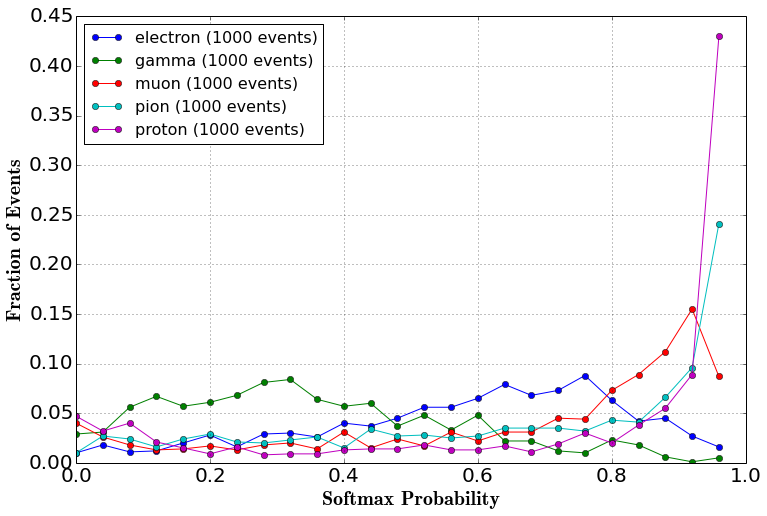# Tutorial 06: public data inference (classification)

Posted on Wed 06 December 2017 in misc by Kazuhiro Terao

In this notebook we will run an inference of the network trained in the training example. For data sample we use the same sample used to test the network during the training (practice_test_10k.root can be found at our public dataset). For the setup of this example, we need to prepare practice_test_10k.root in the current directory. Let us make symbolic links.

In :
%%bash
# Preparation: make symbolic links for practice_test_5k.root
PRACTICE_FILE_DIR=../../
ln -sf $PRACTICE_FILE_DIR/practice_test_5k.root ./test.root  We will use the network's weights from the previous training available under weights directory. In : %%bash ls weights/toynet-4999*  weights/toynet-4999.data-00000-of-00001 weights/toynet-4999.index weights/toynet-4999.meta  ## Imports¶ In : from __future__ import print_function from larcv import larcv from larcv.dataloader2 import larcv_threadio import numpy as np import matplotlib import matplotlib.pyplot as plt %matplotlib inline import os,sys,time # tensorflow/gpu start-up configuration os.environ['TF_CPP_MIN_LOG_LEVEL'] = '3' %env CUDA_DEVICE_ORDER=PCI_BUS_ID %env CUDA_VISIBLE_DEVICES=2 import tensorflow as tf  env: CUDA_DEVICE_ORDER=PCI_BUS_ID env: CUDA_VISIBLE_DEVICES=2  ## Configurations¶ In : TUTORIAL_DIR = '..' IO_CONFIG = os.path.join(TUTORIAL_DIR, 'tf/io_inference.cfg') BATCH_SIZE = 250 NUM_CLASS = 5 SNAPSHOT = "weights/toynet-4999"  A lot simpler since we aren't training and not saving logs therefore. But here's a reminder list of what these are. • TUTORIAL_DIR ... points to the top-level directory of the larcv-tutorial repostitory. • IO_CONFIG ... a configuration file for larcv_threadio to read data for testing. • BATCH_SIZE ... a number of images to be loaded in 1 batch. • NUM_CLASS ... a number of classification categories. ## Configure data reader¶ Again simpler compared to the training phase. We just need 1 data reader interface. In : # # Step 0: IO # # for "test" data set test_io = larcv_threadio() # create io interface test_io_cfg = {'filler_name' : 'TestIO', 'verbosity' : 0, 'filler_cfg' : IO_CONFIG} test_io.configure(test_io_cfg) # configure test_io.start_manager(BATCH_SIZE) # start read thread time.sleep(2) # Now we load 1st batch of data. Unlike in the training, enable the flags to store "entry ID" keys. test_io.next(store_entries=True,store_event_ids=True)   setting verbosity 3  ## Definine a network¶ We use the exact same definition as the training notebook! In : # # Step 1: Define network # import tensorflow.contrib.slim as slim import tensorflow.python.platform def build(input_tensor, num_class=4, trainable=True, debug=True): net = input_tensor if debug: print('input tensor:', input_tensor.shape) filters = 32 num_modules = 5 with tf.variable_scope('conv'): for step in xrange(5): stride = 2 if step: stride = 1 net = slim.conv2d(inputs = net, # input tensor num_outputs = filters, # number of filters (neurons) = # of output feature maps kernel_size = [3,3], # kernel size stride = stride, # stride size trainable = trainable, # train or inference activation_fn = tf.nn.relu, # relu scope = 'conv%da_conv' % step) net = slim.conv2d(inputs = net, # input tensor num_outputs = filters, # number of filters (neurons) = # of output feature maps kernel_size = [3,3], # kernel size stride = 1, # stride size trainable = trainable, # train or inference activation_fn = tf.nn.relu, # relu scope = 'conv%db_conv' % step) if (step+1) < num_modules: net = slim.max_pool2d(inputs = net, # input tensor kernel_size = [2,2], # kernel size stride = 2, # stride size scope = 'conv%d_pool' % step) else: net = tf.layers.average_pooling2d(inputs = net, pool_size = [net.get_shape()[-2].value,net.get_shape()[-3].value], strides = 1, padding = 'valid', name = 'conv%d_pool' % step) filters *= 2 if debug: print('After step',step,'shape',net.shape) with tf.variable_scope('final'): net = slim.flatten(net, scope='flatten') if debug: print('After flattening', net.shape) net = slim.fully_connected(net, int(num_class), scope='final_fc') if debug: print('After final_fc', net.shape) return net  ## Build the network¶ Build the network. Unlike the training example notebook, we don't need accuracy and loss since we won't be monitoring those. Instead we will add an operation to compute softmax probability per class. In : # # Step 2: Build network + define loss & solver # # retrieve dimensions of data for network construction dim_data = test_io.fetch_data('test_image').dim() # define place holders data_tensor = tf.placeholder(tf.float32, [None, dim_data * dim_data * dim_data], name='image') data_tensor_2d = tf.reshape(data_tensor, [-1,dim_data,dim_data,dim_data],name='image_reshape') # build net net = build(input_tensor=data_tensor_2d, num_class=NUM_CLASS, trainable=False, debug=False) # Define softmax softmax_op = tf.nn.softmax(logits=net)  ## Load the network's weights¶ Here is a key difference from the training notebook. Instead of using tf.train.Saver to save the network's weights, we use it to load the trained network's weights this time. In : # # Step 3: tensorflow IO # # Create a session sess = tf.InteractiveSession() # Load weights saver = tf.train.Saver() saver.restore(sess, SNAPSHOT)  INFO:tensorflow:Restoring parameters from weights/toynet-4999  ## Inference 1¶ We will create a csv file that contains the network's output (softmax probability per particle). First, let's follow the format used for the data challenge. In : csv_filename = 'tutorial06-classification-inference1.csv' fout=open(csv_filename,'w') fout.write('entry,run,subrun,event,prediction,probability\n') ctr = 0 num_events = test_io.fetch_n_entries() while ctr < num_events: test_data = test_io.fetch_data('test_image').data() feed_dict = { data_tensor : test_data } softmax_batch = sess.run(softmax_op, feed_dict=feed_dict) processed_events = test_io.fetch_event_ids() processed_entries = test_io.fetch_entries() for j in xrange(len(softmax_batch)): softmax_array = softmax_batch[j] entry = processed_entries[j] event_id = processed_events[j] prediction = np.argmax(softmax_array) prediction_prob = softmax_array[prediction] data_string = '%d,%d,%d,%d,%d,%g\n' % (entry,event_id.run(),event_id.subrun(),event_id.event(), prediction, prediction_prob) fout.write(data_string) ctr += 1 if ctr == num_events: break if ctr == num_events: break test_io.next(store_entries=True,store_event_ids=True) fout.close()  ## Results 1¶ Let's load and plot softmax distribution for different classes. In : import pandas as pd df = pd.read_csv(csv_filename) df.describe()  Out: entry run subrun event prediction probability count 5000.000000 5000.0 5000.000000 5000.000000 5000.000000 5000.000000 mean 2499.500000 1.0 16.801400 1630.542600 1.992000 0.742043 std 1443.520003 0.0 3.991384 399.403894 1.449675 0.188102 min 0.000000 1.0 11.000000 1001.000000 0.000000 0.249298 25% 1249.750000 1.0 14.000000 1319.000000 0.000000 0.585518 50% 2499.500000 1.0 17.000000 1603.000000 2.000000 0.753465 75% 3749.250000 1.0 19.000000 1869.000000 3.000000 0.919953 max 4999.000000 1.0 28.000000 2800.000000 4.000000 1.000000 So we got 5000 events in this sample. 5 particle types are represented by labels 0 to 4. Remember what these indexes correspond to? In : %%bash -s "$IO_CONFIG"
cat \$1 | grep PdgClassList

      PdgClassList: [11,22,13,211,2212]


Right, so it's [11,22,13,211,2212], or in string labels [electron,gamma,muon,pion,proton]. Just as a quick exercize, let's histogram the softmax-probability distribution per predicted label.

In :
import matplotlib
import matplotlib.pyplot as plt
%matplotlib inline

particles = ['electron','gamma','muon','pion','proton']

fig,ax = plt.subplots(figsize=(12,8),facecolor='w')
for index, particle in enumerate(particles):
# Query subset of data predicted to be "this" particle
sub_df = df.query('prediction==%d' % index)
# Make a binned distribution (and scatter-plot it since 5 histogram overlay is hard to see)
hist, _ = np.histogram(sub_df.probability.values, bins=25, range=(0.,1.))
# Plot
label = '%s (%d events)' % (particle,sub_df.index.size)
plt.plot(np.arange(0.,1.,1./25.),hist, label=label, marker='o')
# Decoration!
plt.tick_params(labelsize=20)
plt.xlabel('Softmax Probability',fontsize=20,fontweight='bold',fontname='Georgia')
plt.ylabel('Fraction of Events',fontsize=20,fontweight='bold',fontname='Georgia')
leg=plt.legend(fontsize=16,loc=2)
leg_frame=leg.get_frame()
leg_frame.set_facecolor('white')
plt.grid()
plt.show()It shows that the network has high confidence when it's predicitng a proton but not quite certain when it comes to conclude electron or gamma. Probably pion is also having a hard time though it is hard to even see it here.

We can study more about our network's performance if we have truth information (i.e. label). Luckily we are not working on the challenge data but just test data which contains truth information. So let's store the truth label to project onto the correct label.

## Inference 2¶

All we want is to store additional variable in the csv file. But let's just do the loop one more time because it is instructive though it's slow. Take this opportunity to learn how to set the file reader to read a specific entry.

In :
# First we need to purge the storage buffer
test_io.purge_storage()   # remove all data stored in buffer (since we'll re-read)
test_io.set_next_index(0) # explicitly set the next read happens @ index=0 (first event)

test_io.start_manager(BATCH_SIZE)
test_io.next(store_entries=True,store_event_ids=True)


Let's run the loop again! We'll create a separate csv file.

In :
csv_filename = 'tutorial06-classification-inference2.csv'
fout=open(csv_filename,'w')
fout.write('entry,run,subrun,event,prediction,prediction_probability,label,label_probability\n')
ctr = 0
num_events = test_io.fetch_n_entries()
while ctr < num_events:

test_data  = test_io.fetch_data('test_image').data()
test_label = test_io.fetch_data('test_label').data()
feed_dict = { data_tensor  : test_data }

softmax_batch     = sess.run(softmax_op, feed_dict=feed_dict)
processed_events  = test_io.fetch_event_ids()
processed_entries = test_io.fetch_entries()

for j in xrange(len(softmax_batch)):
softmax_array = softmax_batch[j]
entry         = processed_entries[j]
event_id      = processed_events[j]

prediction      = np.argmax(softmax_array)
prediction_prob = softmax_array[prediction]
label           = np.argmax(test_label[j])
label_prob      = softmax_array[label]

data_string = '%d,%d,%d,%d,%d,%g,%d,%g\n' % (entry,
event_id.run(), event_id.subrun(), event_id.event(),
prediction, prediction_prob,
label,label_prob)
fout.write(data_string)

ctr += 1
if ctr == num_events:
break
if ctr == num_events:
break

test_io.next(store_entries=True,store_event_ids=True)

fout.close()


This time, we will group events by its truth label and plot the label's softmax probability:

In :
df = pd.read_csv(csv_filename)
particles = ['electron','gamma','muon','pion','proton']

fig,ax = plt.subplots(figsize=(12,8),facecolor='w')
for index, particle in enumerate(particles):
# Query subset of data predicted to be "this" particle
sub_df = df.query('label==%d' % index)

# Make a binned distribution (and scatter-plot it since 5 histogram overlay is hard to see)
hist, _ = np.histogram(sub_df.label_probability.values,
bins=25, range=(0.,1.),
weights=[1./sub_df.index.size] * sub_df.index.size)
# Plot
label = '%s (%d events)' % (particle,sub_df.index.size)
plt.plot(np.arange(0.,1.,1./25.), hist, label=label, marker='o')
# Decoration!
plt.tick_params(labelsize=20)
plt.xlabel('Softmax Probability',fontsize=20,fontweight='bold',fontname='Georgia')
plt.ylabel('Fraction of Events',fontsize=20,fontweight='bold',fontname='Georgia')
leg=plt.legend(fontsize=16,loc=2)
leg_frame=leg.get_frame()
leg_frame.set_facecolor('white')
plt.grid()
plt.show()This plot is somewhat similar to the one we plotted earlier using prediction softmax probability. Probably the most notable is that blue (electron) and green (gamma) got worse (i.e. peak moved toward left and/or peak smeared). Is a network doing somewhat random guess on them? Possibly for gamma, maybe better than random for electron.

But thinking from physics perspective, we realize that electron and gamma share great similarity: they are both EM-shower particles. This means their geometrical shapes are quite alike, and are easily distinguishable from the rest of partciles (i.e. none-EM-shower, or track particles). Having the ground-truth allows us to check on this assumption.

In :
# Query EM-Shower (electron or gamma ray) particles
emshower = df.query('label in [0,1]').index.size
# Count how many of them were selected right
emshower_correct = df.query('label in [0,1] and prediction in [0,1]').index.size
print('EM-Shower correct fraction:',emshower_correct,'/',emshower,'=',float(emshower_correct)/emshower*100.,'[%]')

# Repeat for track (i.e. non-EM-shower) particles
track = df.query('label not in [0,1]').index.size
# Count how many of them were selected right
track_correct = df.query('label not in [0,1] and prediction not in [0,1]').index.size
print('Track correct fraction:',track_correct,'/',track,'=',float(track_correct)/track*100.,'[%]')

EM-Shower correct fraction: 1722 / 2000 = 86.1 [%]
Track correct fraction: 2958 / 3000 = 98.6 [%]


... so indeed the trained network seems to distinguish two set of geometrical shapes (i.e. EM-shower and track particles) quite well. At the same time, this means the network is having very hard time distinguishing electron from gamma and vice versa.

Coming back to the main point, in this notebook we exercised how to run a simple inference using larcv_threadio. So what is not simple inference? In the next tutorial, we go over how to store the output into larcv format data file in the case of running a semantic segmentation network or SSNet. In this example the "output" from the network was just an array of floats (i.e. softmax probability). But in the case of SSNet, the output is essentially an image with many channels, which can be stored just like input image into a larcv file.